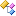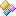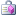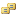NationalInstruments.Analysis.Math Namespace

Measurement Studio 2012 for Visual Studio 2010 Help

Edition Date: August 2012

Part Number: 372636F-01

»View Product InfoDownload Help (Windows Only)

Classes

ClassDescriptionArrayOperationProvides a set of methods that perform array operations.Calculus Provides a set of methods for integration. For differentiation, refer to DifferentiateComplexMath Provides a set of methods for common mathematical functions on complex numbers.CurveFit Provides a set of methods that fit data to mathematical functions and interpolate unknown points from a known data set.LinearAlgebra Provides a set of methods that perform linear algebra operations.Probability Provides a set of methods that calculate error functions, probability distribution functions and their inverses, and rate contingency tables.Roots Provides a set of methods that find the zeroes of mathematical functions.SpecialFunctionsProvides a set of methods that implement special functions.Statistics Provides a set of methods that perform statistical operations on data.

Structures

StructureDescriptionStatistics.Anova1WayOutput Returns the output of the one-way analysis of variance.Statistics.Anova2WayOutput Returns the output of the two-way analysis of variance.Statistics.Anova3WayOutput Returns the output of the three-way analysis of variance.

Delegates

DelegateDescriptionModelFunctionCallback Specifies the callback function to be used in NonLinearFit.

Enumerations

EnumerationDescriptionAnovaModel Specifies whether a fixed or random effect is to be used in the analysis of variance.AutocorrelationMatrixMethod Specifies the method used to compute the autocorrelation matrix.BalanceMethod Specifies how to balance a matrix.BestFitAlgorithm Specifies the algorithm to be used in solving the multiple linear regression model.EigenMatrixType Specifies the type of input matrix for the EigenValueVector method.EigenVectorSide Specifies the type of generalized eigenvectors of the matrix pair (A,B).FitMethod The fit method used by the curve-fitting methods.IntegrationMethod Specifies the IntegrationMethod used for numeric integration.LogarithmType The base of the logarithm used by the LogarithmFit and LogarithmFitInPlace curve-fitting methods.MatrixType Specifies the type of the input matrix.NormType Specifies the type of norm used to calculate the norm of the input matrix.PolynomialFitAlgorithm Specifies the fit algorithm used by the specified PolynomialFit or PolynomialFitInPlace curve-fitting method.QRAlgorithm Specifies the algorithm to be used for QR factorization.RangeType Specifies the type of range used by the RemoveOutliersUsingRange method.ReorderMechanism Specifies how to reorder the computed eigenvalues and corresponding Schur vectors.SizeOption Specifies the size option for the SvdFactorization and QRFactorization methods.VectorNormType Specifies the norm type used to calculate the norm of the input vector.